ConicBundle
ConicBundle::BundleProxObject Class Referenceabstract

abstract interface that allows to use different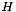-norms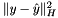with a positive definite matrixin the proximal term of the augmented model of ConicBundle::BundleSolver. More...

#include <BundleProxObject.hxx>

Inheritance diagram for ConicBundle::BundleProxObject:Public Member Functions

BundleProxObject (VariableMetricSelection *vp=0, bool local_scaling=false, bool bounds_scaling=false, CBout *cb=0, int cbincr=-1)
default constructor, switching on dynamic scaling only works for classes with corresponding support

virtual void set_weightu (CH_Matrix_Classes::Real weightu)=0
in future computations the following weight must be included in the quadratic term (replacing the previous one)

virtual void set_short_QPsteps (CH_Matrix_Classes::Integer shortQPst)
may be used to indicate seemingly conservative step sizes possibly due to the quadratic term

virtual CH_Matrix_Classes::Integer get_short_QPsteps ()
retrieves the number of conservative step sizes possibly due to the quadratic term passed on to this

int apply_factor (CH_Matrix_Classes::Real f)
allows AFTModel and SumModel to accumulate a compensation factor for tracing the effects of recursive applications of function_factor in update_model (does not affect H but can be retrieved by get_factor() for this purpose)

CH_Matrix_Classes::Real get_factor ()
returns the current accumulated compensation factor by which H would need to be scaled in order to reflect the curvature relative to the current function without function_factor;

virtual CH_Matrix_Classes::Real get_weightu () const =0
return the current weight incorporated in the quadratic term

virtual CH_Matrix_Classes::Real get_term_corr (void) const
return a correction factor for termination precision if the quadratic term is strong

virtual CH_Matrix_Classes::Real norm_sqr (const CH_Matrix_Classes::Matrix &B) const =0
return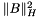virtual CH_Matrix_Classes::Real dnorm_sqr (const MinorantPointer &B) const =0
return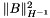virtual bool is_DLR () const =0
return true if H is of the form diagonal matrix plus Gram matrix of a low rank matrix

virtual int add_H (CH_Matrix_Classes::Symmatrix &big_sym, CH_Matrix_Classes::Integer start_index=0) const =0
add H to the dense symmetric matrix as a principal submatrix starting at position start_index

virtual CH_Matrix_Classes::Matrixadd_Hx (const CH_Matrix_Classes::Matrix &x, CH_Matrix_Classes::Matrix &outplusHx, CH_Matrix_Classes::Real alpha=1.) const =0
addto outplusHx and return this

virtual CH_Matrix_Classes::Matrixapply_Hinv (CH_Matrix_Classes::Matrix &x) const =0
returns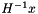, possibly transformed by some AffineFunctionTransformation More...

virtual void get_precond (CH_Matrix_Classes::Matrix &D, const CH_Matrix_Classes::Matrix *&Vp) const =0
return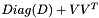which either equals $H$ exactly (iff is_DLR() returns true) or, if not, serves as an approximation hopefully suitable for preconditioning. If $V$ is available it may be large, so return only a pointer to avoid copying. If no $V$ is available, then put Vp==0 on exit.

virtual int compute_QP_costs (CH_Matrix_Classes::Symmatrix &Q, CH_Matrix_Classes::Matrix &d, CH_Matrix_Classes::Real &offset, const MinorantPointer &constant_minorant, const MinorantBundle &bundle, const CH_Matrix_Classes::Matrix &center_y, const MinorantPointer &groundset_minorant, CH_Matrix_Classes::Indexmatrix *yfixed)=0
Compute the dual QP costs Q, d, and the constant offset to the bundle subproblem. More...

virtual int update_QP_costs (CH_Matrix_Classes::Symmatrix &delta_Q, CH_Matrix_Classes::Matrix &delta_d, CH_Matrix_Classes::Real &delta_offset, const MinorantPointer &constant_minorant, const MinorantBundle &bundle, const CH_Matrix_Classes::Matrix &center_y, const MinorantPointer &groundset_minorant, const MinorantPointer &delta_groundset_minorant, const CH_Matrix_Classes::Indexmatrix &delta_index, CH_Matrix_Classes::Indexmatrix *yfixed)=0
computes the update of the dual QP cost terms d and offset returned by compute_QP_costs() for changes in the ground set aggregate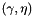and in yfixed. More...

virtual int apply_modification (const GroundsetModification &gsmdf)=0
if the BundleSolver is called to modify the groundset it also calls this More...

virtual BundleProxObjectprojected_clone (const CH_Matrix_Classes::Indexmatrix &indices)=0
in order to allow for fixed variables, this generates a clone restricted to the given indicesPublic Member Functions inherited from ConicBundle::VariableMetric
virtual ~VariableMetric ()
virtual destructor

VariableMetric (VariableMetricSelection *vp=0, bool use_loc_metric=false, bool use_bnds_scaling=false, CBout *cbo=0, int cbinc=-1)
default constructor; if vp is not zero, ownership of *vp is passed over to *this and *this will delete vp

VariableMetricSelectionget_variable_metric_selection () const
returns 0 or an available VariableMetricSelection object, that may be employed for computing a variable metric term in accordance with the value of use_local_metric

void set_variable_metric_selection (VariableMetricSelection *vp=0)
sets use_variable_metric; the object passed is then owned by this

virtual bool supports_dense_variable_metric () const
returns true if add_dense_variable_metric() is supported

virtual bool supports_lowrank_variable_metric () const
returns true if add_variable_metric() does not ignore the low rank argument vecH

virtual bool supports_diagonal_variable_metric () const
returns true if add_variable_metric() does not ignore the diagonal argument diagH

bool employ_variable_metric () const
returns true if some dynamic scaling is supported and switched on

virtual int apply_variable_metric (VariableMetricModel *, VariableMetricModel *, const CH_Matrix_Classes::Matrix &, CH_Matrix_Classes::Integer, const CH_Matrix_Classes::Matrix &, bool, CH_Matrix_Classes::Real &, CH_Matrix_Classes::Real, const CH_Matrix_Classes::Indexmatrix *=0)
the BundleSolver starts an update of this by dynamic scaling by calling this in every step; negative parameters give no preferences, but the global aggregate (groundset+model) has to be provided and if descent_step==false the scaling may only increase

adds a suitable modification of symH (symH may be modified in this) to the scaling matrix H More...

virtual int add_variable_metric (CH_Matrix_Classes::Matrix &, CH_Matrix_Classes::Matrix &)
adds (a suitable modification of) Diag(diagH)+vecH*transpose(vecH) to the scaling matrix H (either matrix may be modified in this) More...

virtual int push_aft (const AffineFunctionTransformation *)
this AffineFunctionTransformation has to be used before applying Hinv in

virtual int pop_aft ()
removes the top most aft (without deleting it!)

bool get_use_bounds_scaling () const
returns use_bounds_scaling

void set_use_bounds_scaling (bool bounds_scaling)
sets use_bounds_scaling

virtual bool supports_diagonal_bounds_scaling () const
if the respective implementation supports a diagonal bounds scaling heuristic, the following routine has to return true; see also diagonal_scaling_heuristic_update()

bool employ_diagonal_bounds_scaling () const
if the respective implementation supports a diagonal bounds scaling heuristic, the following routine has to return true; see also diagonal_bounds_scaling_update()

virtual int diagonal_bounds_scaling_update (const CH_Matrix_Classes::Matrix &)
if supported, D_update has to contain nonnegative numbers that are permanently added to the diagonal here. More...

bool get_use_local_metric () const
returns use_local_metric

void set_use_local_metric (bool local_metric)
sets use_local_metricPublic Member Functions inherited from ConicBundle::CBout
virtual void set_out (std::ostream *out=0, int print_level=1)
Specifies the output level (out==NULL: no output at all, out!=NULL and level=0: errors and warnings, level>0 increasingly detailed information) More...

virtual void set_cbout (const CBout *cb, int incr=-1)
Specifies the output level relative to the given CBout class. More...

void clear_cbout ()
reset to default settings (out=0,print_level=1)

CBout (const CBout *cb=0, int incr=-1)
calls set_cbout

CBout (std::ostream *outp, int pl=1)
initialize correspondingly

CBout (const CBout &cb, int incr=0)
copy constructor

virtual bool cb_out (int pl=-1) const
Returns true if out!=0 and (pl<print_level), pl<0 should be used for WARNINGS and ERRORS only, pl==0 for usual output.

std::ostream & get_out () const
If cb_out() returned true, this returns the output stream, but it will abort if called with out==0.

std::ostream * get_out_ptr () const
returns the pointer to the output stream

int get_print_level () const
returns the print_level

virtual int mfile_data (std::ostream &out) const
writes problem data to the given outstreamPublic Member Functions inherited from ConicBundle::QPSolverProxObject
virtual ~QPSolverProxObject ()
virtual destructor

Protected Attributes

CH_Matrix_Classes::Real factor
used to accumulate a compensation factor for function_factor; this factor is not included in H but can be applied externally via get_factor() if required

CH_Matrix_Classes::Integer short_QPsteps
the QP may signal short steps that seem due to the quadratic term by setting this counter via set_short_QPsteps()

Static Protected Attributes

static const CH_Matrix_Classes::Integer xdim_threshold = 100
constant for possible use if QP coefficients are to be computed in parallel (still purely experimental)

Detailed Description

abstract interface that allows to use different-normswith a positive definite matrixin the proximal term of the augmented model of ConicBundle::BundleSolver.

ConicBundle::BundleIdProx is the identitiy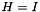. There are further variants for diagonal scaling, low rank scaling or full scaling by a positive definite matrix.

◆ apply_Hinv()

 virtual CH_Matrix_Classes::Matrix& ConicBundle::BundleProxObject::apply_Hinv ( CH_Matrix_Classes::Matrix & x ) const
pure virtual

returns, possibly transformed by some AffineFunctionTransformation

If a stack of instances of AffineFunctionTransformation has been added by push_aft() and A_1 to A_k are on the stack with A_k on top, then it returns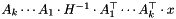Implements ConicBundle::QPSolverProxObject.

Referenced by get_term_corr().

◆ apply_modification()

 virtual int ConicBundle::BundleProxObject::apply_modification ( const GroundsetModification & gsmdf )
pure virtual

if the BundleSolver is called to modify the groundset it also calls this

The task is then to update the diagonal scaling information appropriately so that dimensions match and the proximal term makes sense for the modified problem.

Referenced by get_term_corr().

◆ compute_QP_costs()

 virtual int ConicBundle::BundleProxObject::compute_QP_costs ( CH_Matrix_Classes::Symmatrix & Q, CH_Matrix_Classes::Matrix & d, CH_Matrix_Classes::Real & offset, const MinorantPointer & constant_minorant, const MinorantBundle & bundle, const CH_Matrix_Classes::Matrix & center_y, const MinorantPointer & groundset_minorant, CH_Matrix_Classes::Indexmatrix * yfixed )
pure virtual

Compute the dual QP costs Q, d, and the constant offset to the bundle subproblem.

Let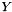denote the (convex) feasible ConicBundle::Groundset and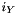its indicator function, let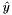be the center of stability given by center_y, let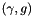be the aggregate ground set minorant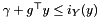described by gs_subg_offset and gs_subg, letdenote the positive definite scaling matrix with weight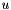given by this class, and let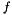denote the objective for which ConicBundle::BundleModel holds the model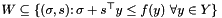, which is assumed to be described in the form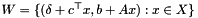for some compact convex set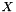of dimension xdim. The routine computes the cost coefficients for the convex quadratic problem over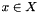corresponding to the saddle problem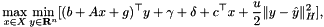This is indeed a convex QP in x because for each x the inner minimization over y is unconstrained and can be solved explicitly. So this gives rise to a problem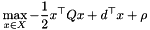How to compute the cost coefficients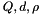efficiently depends on the structural properties ofand therefore the costs are computed in this class.

The data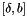is provided by the offset constant minorant (its offset is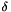, its linear part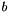), likewise the columns of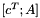are provided by the xdim minorants of the bundle anddescribes the admissible convex combinations which are not needed here.andare the quadratic term and the weight, the latter is set in set_weightu(). The cost coefficients are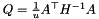, d=...

If some of the design variables are at their bounds and likely to only move out of bounds next they might be required to stay fixed by the calling routine and thus may not contribute to the cost coefficients of the dual QP other than by their contribution to the constant offset. These variables are indicated by a positive (nonzero) value in yfixed.

Parameters
 [out] Q (CH_Matrix_Classes::Symmatrix&) the quadratic cost term to be computed by this routine [out] d (CH_Matrix_Classes::Matrix&) the linear cost term to be computed by this routine [out] offset (CH_Matrix_Classes::Real&) the constant cost offset term to be computed by this routine [in] constant_minorant (const MinorantPointer&) together with the groundset_minorant its offset will yield, and its linear part), [in] bundle (const MinorantBundle&) their offsets form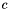, their linear parts the columns of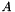. [in] center_y (const CH_Matrix_Classes::Matrix&) the current center of stability of the bundle method, it is needed for computing linear cost term and constant coefficient [in] groundset_minorant (const MinorantPointer&) this is the aggregate subgradient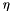of the ground set it acts like a shift of the vector b forming the linear cost term for the y variables. [in,out] yfixed (CH_Matrix_Classes::Indexmatrix*) if not NULL, on input the values of yfixed[i] are 0 if y_i us to be treated as a normal/non-fixed variable 1 if y_i is to be treated as a fixed value again 2 if y_i is newly fixed for the first time on output the last group, those with value 2 have to be set to 1 so that only values 0 and 1 remain
Returns
• 0 on success
• 1 on failure

Referenced by get_term_corr().

◆ update_QP_costs()

 virtual int ConicBundle::BundleProxObject::update_QP_costs ( CH_Matrix_Classes::Symmatrix & delta_Q, CH_Matrix_Classes::Matrix & delta_d, CH_Matrix_Classes::Real & delta_offset, const MinorantPointer & constant_minorant, const MinorantBundle & bundle, const CH_Matrix_Classes::Matrix & center_y, const MinorantPointer & groundset_minorant, const MinorantPointer & delta_groundset_minorant, const CH_Matrix_Classes::Indexmatrix & delta_index, CH_Matrix_Classes::Indexmatrix * yfixed )
pure virtual

computes the update of the dual QP cost terms d and offset returned by compute_QP_costs() for changes in the ground set aggregateand in yfixed.

The update of the groundset aggregate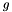is given by delta_gs_subg and delta_index, more precisely, old_gs_subg[delta_index[i]]=gs_subg[delta_index[i]]-delta_gs_subg[i], where the current groundset aggregate gs_subg is the input paramter. delta_gs_subg_offset gives the change of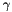.

The entries of yfixed have the same meaning as in compute_QP_costs(): if yfixed[i]==0, variable y[i] is treated normally (not fixed); for yfixed[i]==1 the value was already considered fixed at center_y[i] in the computations before so it causes no changes at all; for yfixed[i]==2 the value is considered newly fixed at center_y[i], so its influence in the quadratic cost term Q and the linear term d have to be corrected (this case is the only reason for having delta_Q in the list of arguments). After this correction, put yfixed[i]=1 to signal that in the QP costs coordinate i is considered as fixed.

Parameters
 [out] delta_Q (CH_Matrix_Classes::Symmatrix&) the change in the quadratic cost term to be computed by this routine [out] delta_d (CH_Matrix_Classes::Matrix&) the change in linear cost term to be computed by this routine [out] delta_offset (CH_Matrix_Classes::Real&) the change in the constant cost offset term to be computed by this routine [in] constant_minorant (const MinorantPointer&) must be identical to the one used in compute_QP_costs [in] bundle (const CH_Matrix_Classes::Integer) must be identical to the one used in compute_QP_costs [in] center_y (const CH_Matrix_Classes::Matrix&) the current center of stability of the bundle method, [in] groundset_minorant (const MinorantPointer&) this is the aggregate subgradientof the ground set [in] delta_groundset_minorant (const MinorantPointer&) this is the delta added to the previous value of the groundset aggregate to get the current aggregate subgradient=groundset_minorant of the ground set [in] delta_index (const CH_Matrix_Classes::Indexmatrix&) holds the inidices where groundset_minorant or yfixed changed [in,out] yfixed (CH_Matrix_Classes::Indexmatrix*) if not NULL, on input the values of yfixed[i] are 0 if y_i us to be treated as a normal/non-fixed variable 1 if y_i is to be treated as a fixed value again 2 if y_i is newly fixed for the first time on output the last group, those with value 2 have to be set to 1 so that only values 0 and 1 remain
Returns
• 0 on success
• 1 on failure